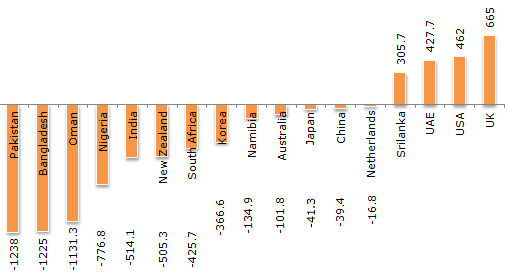# Data Interpretation - Bar Charts - Discussion

Discussion Forum : Bar Charts - Bar Chart 17 (Q.No. 2)
Directions to Solve

The total of the first three deficit countries (in Rs. crores) = 3594.3. The total of next five deficit countries (in Rs. crores) = 2588.5. The total of the last five deficit countries (in Rs. crores) = 334.2.

Cumulative Trade Deficit/Surplus of Countries for the Year 2006 - 2007 (All figures in Rs. Crores).2.
The average of the total deficit of the middle five deficit is closest to the deficit of which country ?
Korea
South Africa
UAE
None of these
Explanation:

The middle five deficit countries are India, New Zealand, South Africa, Korea and Namibia.

Their average deficit is (1946.6/5) = 389.32. Korea's deficit is close to this value.

Discussion:
3 comments Page 1 of 1.

Deepu said:   4 years ago
First, know the deficit meaning is too small in bar chart below zero are deficit. Total deficit countries are 13. They asked the middle 5 deficit countries. I am dividing 13 into 4+5+4. The middle one is 5.

Those five deficit countries are India, New Zealand, South Africa, Korea and Namibia(Average of 5 deficit countries is 514.1+505.3+425.7+366.6+134.9=1946.6

The average of middle five deficit countries is = 1946.6/5 = 389.32 This is nearest to Korea. So option A is correct
(2)

Thongs said:   5 years ago
For me, I got 1534. while adding all the deficit of the middle five countries in which is divided by 5 and got the answer 306.86(1534. 3/5=306.86) and I considered it as nearest to Korea. Please correct me, if I am wrong.

Toka said:   5 years ago
How come 1946.6?

While adding all five deficit. It comes -2588.5. (2588.2/5)=517.7. Its near to India.

Anyone explain me?